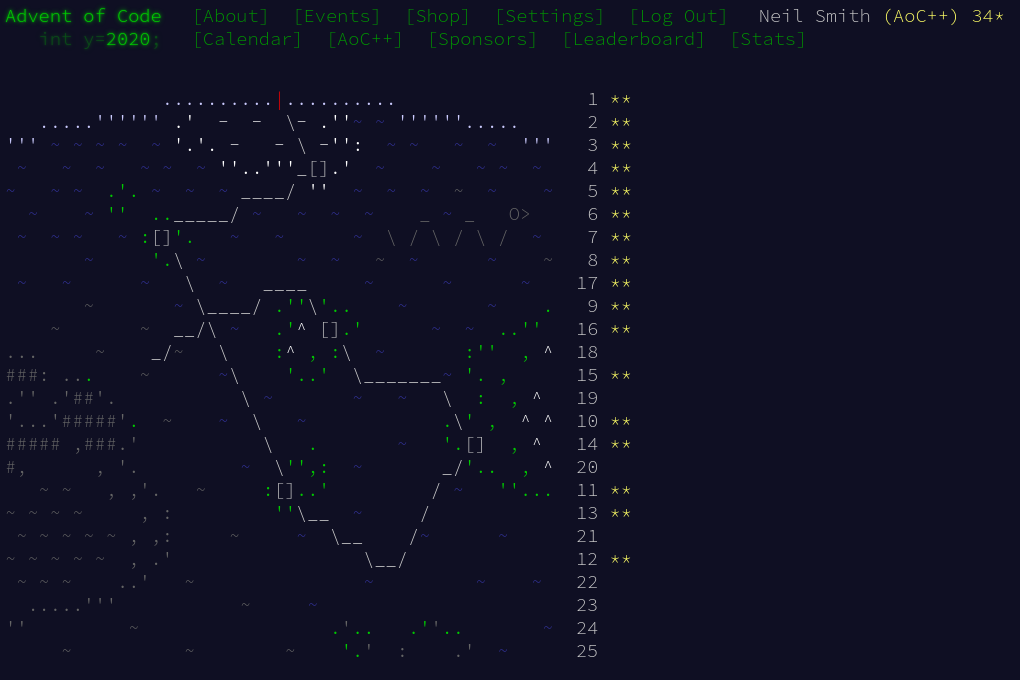December 26, 2020

# Advent of Code 2020 day 17

Cellular automata and typeclassesFor day 17, I drew on last year's Advent of Code, mainly day 12 (for typeclasses) and day 24 (for running the cellular automaton).

## Part 1

First up, data structures. I chose a V3 vector from the Linear library to represent a position, and a Set of them to represent the active cubes.

Reading the grid is iterating over all cells in the grid, filling the set of active cubes as we go.

type Coord = V3 Int -- x, y, z
type Grid = S.Set Coord

readGrid :: String -> IO Grid
let grid = lines gs
let isActive x y = (grid!!y)!!x == '#'
let maxX = length (head grid) - 1
let maxY = length grid - 1
return $S.fromList [ V3 x y 0 | x <- [0..maxX], y <- [0..maxY], isActive x y]  To update the automaton one step, I check every member of the grid to see if it survives. I also find the "empties", the inactive cubes adjacent to an active cube, and check if any of them become active. update :: Grid -> Grid update grid = S.union (S.filter (cubeSurvives grid) grid) (S.filter (cubeBorn grid) empties) where empties = (S.foldr mergeEmpties S.empty grid) S.difference grid mergeEmpties cell acc = S.union acc$ neighbourSpaces cell


An inactive cube becomes active (is born) if it has three neighbours; an active cube remains active if it has two or three neighbours.

cubeSurvives :: Grid -> Coord -> Bool
cubeSurvives grid cell = alive && (nNbrs == 2 || nNbrs == 3)
where alive = cell S.member grid
nNbrs = countOccupiedNeighbours cell grid

cubeBorn :: Grid -> Coord -> Bool
cubeBorn grid cell = dead && (nNbrs == 3)
where dead = cell S.notMember grid
nNbrs = countOccupiedNeighbours cell grid


Both the rule, and the indentification of the empties, require finding the neighbours of a cell, and possibly counting how many neighbours are active.

neighbourSpaces :: Coord -> Grid
neighbourSpaces here = S.map (here ^+^) nbrs
where nbrs = S.fromList [ V3 dx dy dz
| dx <- [-1, 0, 1]
, dy <- [-1, 0, 1]
, dz <- [-1, 0, 1]
, (dx, dy, dz) /= (0, 0, 0)]

countOccupiedNeighbours :: Coord -> Grid -> Int
countOccupiedNeighbours cell grid =
S.size $S.intersection grid$ neighbourSpaces cell


Finally, the simulation runs by iterating update and dropping the unneeded states.

main =
let finalGrid = head $drop 6$ iterate update grid0
print $S.size finalGrid  ## Part 2 This is exactly the same as part 1, except on a 4-d grid rather than a 3-d grid. The only parts of the code that explicitly refer to the dimensions are the grid construction, and the generation of the neighbours. It seems very wasteful to replicate all the code just to use different functions at the bottom of the call stack. Polymorphism to the rescue! Rather than having Coord as a concrete type, I use it as typeclass, with instances for the 3-d and 4-d cases. I split the neighbourSpaces function into two parts: the mapping of (here ^+^) and the creation of the set of neighbourCells of the origin.(I also need to define a new operator, ^+^^, to add Coords.) class (Num a, Ord a) => Coord a where (^+^^) :: a -> a -> a neighbourCells :: S.Set a instance Coord (V3 Int) where x ^+^^ y = x ^+^ y neighbourCells = S.fromList [ V3 dx dy dz | dx <- [-1, 0, 1] , dy <- [-1, 0, 1] , dz <- [-1, 0, 1] , (dx, dy, dz) /= (0, 0, 0) ] instance Coord (V4 Int) where x ^+^^ y = x ^+^ y neighbourCells = S.fromList [ V4 dx dy dz dw | dx <- [-1, 0, 1] , dy <- [-1, 0, 1] , dz <- [-1, 0, 1] , dw <- [-1, 0, 1] , (dx, dy, dz, dw) /= (0, 0, 0, 0)  The rest of the code stays the same, apart from the use of the new operator in neighbourSpaces and a few changes in types to use the new class. neighbourSpaces :: Coord a => a -> Grid a neighbourSpaces here = S.map (here ^+^^) neighbourCells countOccupiedNeighbours :: Coord a => a -> Grid a -> Int cubeSurvives :: Coord a => Grid a -> a -> Bool  Finally, I need to convert the 3-d grid originally read into a 4-d grid. conv34 :: Grid (V3 Int) -> Grid (V4 Int) conv34 grid = S.map conv34Cell grid conv34Cell (V3 x y z) = V4 x y z 0  The simulations are the same, apart from the dimension conversion. main = do grid0 <- readGrid "data/advent17.txt" print$ part1 grid0
print $part2 grid0 part1 grid0 = S.size finalGrid where finalGrid = head$ drop 6 $iterate update grid0 part2 grid0 = S.size finalGrid where grid4 = conv34 grid0 finalGrid = head$ drop 6 \$ iterate update grid4

You can find the code here or on GitLab. Part 1 is in advent17a.hs, part 2 in advent17.hs.